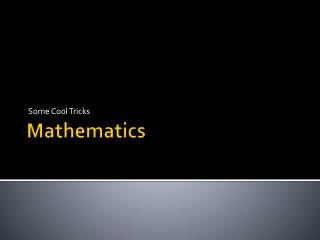DownloadDownload PresentationMathematics

# Mathematics

Télécharger la présentation## Mathematics

- - - - - - - - - - - - - - - - - - - - - - - - - - - E N D - - - - - - - - - - - - - - - - - - - - - - - - - - -
##### Presentation Transcript

1. Some Cool Tricks Mathematics

2. Graphs • We can consider the screen as high school graph paper. • Each sprite or object is located somewhere in the coordinate system.

3. Our Screen • Remember our screen is not like the school mathematical graph paper! The origin is at the top-left! • That means the bottom of our object is at a higher y position than the top!

4. Translation • Moving things around is called translation. • Usually we translate our object to the origin before we perform rotation and scaling.

5. Translation • Move every point in one object to another location.

6. Rotation • For rotation, lets visit the Siggraph web page: https://www.siggraph.org/education/materials/HyperGraph/modeling/mod_tran/2drota.htm, accessed August 2014.

7. Pythagoras • We use Pythagoras to find the distance between two points.

8. Cartesian and Polar Coordinates • Using (x, y) positions is common. • However, you may use (r, Θ) • r = distance from origin • Θ = angle. • Converting between the two systems is easy. • Just remember the name!

9. Rotational Mathematics • Beware, we need a little bit more sophistication to calculate the angle… as this gives us ambiguity.

10. Pythagoras & Collision Detection We can possibly do this with square distances to avoid using expensive sqrt operations. We must be careful to avoid interpenetration as the entities will indefinite collide with each other. • We can use circle to circle collision as an example. • We find the centre of each circle and use Pythagoras to discover the distance between the two. • The equations to the right tell us when there has been a collicsion!

11. Collision Detection Problems • Interpenetration of objects. • Interlocking of objects. • Quantum Tunnelling Effects.

12. Scaling • Once again in scaling we need to translate our object’s origin to the origin of the world, we then perform the scaling operation. • Remember to translate back!

13. Logic • We use logic all the time in coding. • We will use logic for our AI and decision making in games. • We will explicitly cover some rudimentary logic.

14. Truth Tables • And • Or • Not • Xor • Implies • Tautologies—Saying the same thing more than once. • Associative Laws—Order does not matter. • Distributive Laws—We can say things in different ways that mean the same thing. • Identity Laws

15. Truth Tables

16. Associative Laws/Distributive Laws

17. Sets • Sets can be used to make decisions. • Sets indicate belongingness.

18. Set Theory • Venn Diagrams • Union • Intersection • Difference. • Sets • Subsets • Compliment • Universal Set • Identity Laws

19. A Intersect B

20. Multiple Sets • What is in A, B and C? • What is in A? • What is in not A? • Useful for games. • Useful for determining strategies.

21. C++ and Sets • Yes! • std::set! • How awesome is that?

22. Vectors and Matrices • Vectors and matrices are mathematically convenient tools. • They are used in games for graphical mathematics. • So annoying that std::vector is named in such a way to make things a little confusing.

23. Mathematical Problems I like to call this Quantum Tunnelling. We miss the detection of a collision as the bullet passes through the wall.

24. Simple Tricks Bounding box collision detection in 2D. Bounding box collision detection in 2D.

25. Today • Tricks • One’s Compliment

26. Pythagoras • The distance between two points can be calculated using Pythagoras. • This can be used for simple collision detection. • Movie, https://www.youtube.com/watch?v=pVo6szYE13Y, accessed August 2014.

27. Simple Collision Detection • https://www.youtube.com/watch?v=o-OvVep2uSg, accessed August 2014.

28. Analytical Geometry • Analytical Geometry is concerned with points and the relationships between points. • We can find angles between two points to determine interesting relationships.

29. Distance Between Two Points • Useful for determining: • (a) If a collision would take place. • (b) If a collision has taken place.

30. Perpendicular Distance Between Line and Point • Let’s look at the diagrams! • http://www.intmath.com/plane-analytic-geometry/perpendicular-distance-point-line.php, accessed August 2014.

31. Vectors • Vectors can represent acceleration, velocity and displacement and any sort of force, which is essential to any physics orientated game. • It allows for you to calculate paths, trajectories and many aspects of physics within code.

32. Vector • A vector is a point. • For two dimensional mathematics: • (x, y). • These vectors have interesting operations. • They make it easier to do mathematics.

33. Simple Idea • We can simply calculate the resulting vector when combining multiple forces…

34. Matrix • A matrix is a column by row matrix of values. • The matrix has defined operations so we can perform mathematical operations efficiently on a collection of points.

35. Translation Matrix

36. Scaling Matrix

37. Rotation Matrix